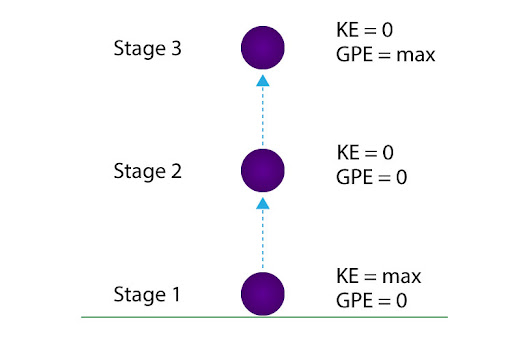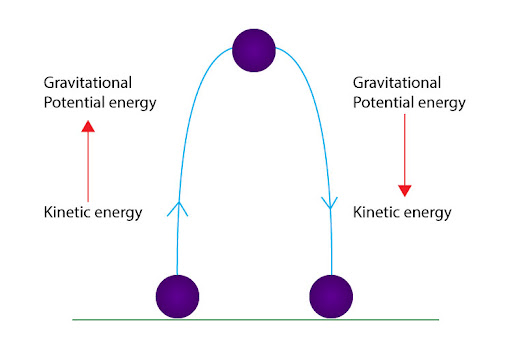# "Describe the transfers of energy occurring when a ball is thrown vertically up in the air and falls back down to Earth, assuming there is no friction from the air."

A: Assuming there’s no friction in air is us assuming that no energy is being converted to an irreversible form of energy like heat. In that regard, for a ball that’s moving vertically up and then back down, there will be two specific types of energy transformations:

Gravitational potential energy - energy stored in an object due to its height
Kinetic energy - energy stored in an object due to its velocity

With that in mind, let us now see how do these forms of energy interchange:At stage 1, the ball has just been launched from the ground. Because it has an initial non-zero velocity, and no height gained, the ball has maximum kinetic energy but no gravitational potential energy.

At stage 2, the ball is mid way. Since it has gained some height, it has gained gravitational potential energy. But since it has slowed down a bit (i.e. it’s velocity has decreased from its initial value), kinetic energy has decreased.

At stage 3 of the motion, the ball stops in mid air, at the highest point of its motion. It has achieved maximum height, meaning it has maximum gravitational potential energy. But since it has stopped momentarily, its kinetic energy decreases to zero.

So as the ball moves up, it’s kinetic energy decreases, while gravitational potential energy increases - in other words, it’s kinetic energy is being converted to gravitational potential energy.

Now you can guess what would happen as the ball falls down; now it is gaining speed and losing height. Meaning it’s kinetic energy is increasing, while gravitational potential energy is increasing. Rephrasing this, we can conclude that as the ball falls down, the gravitational potential energy is being converted to kinetic energy.